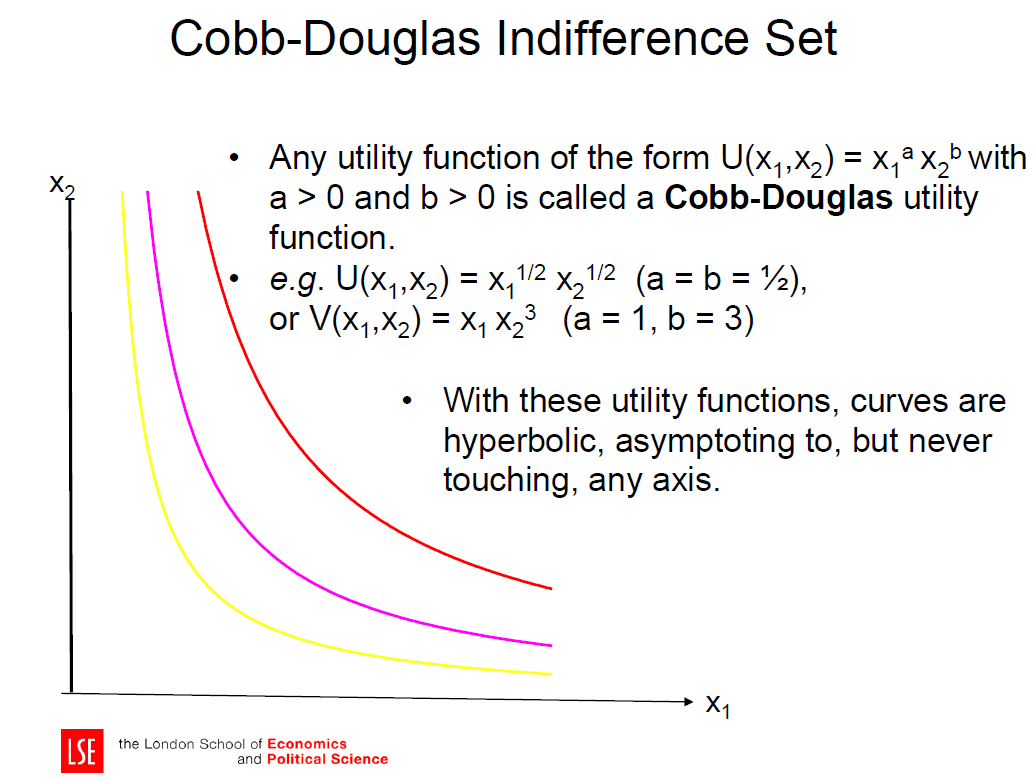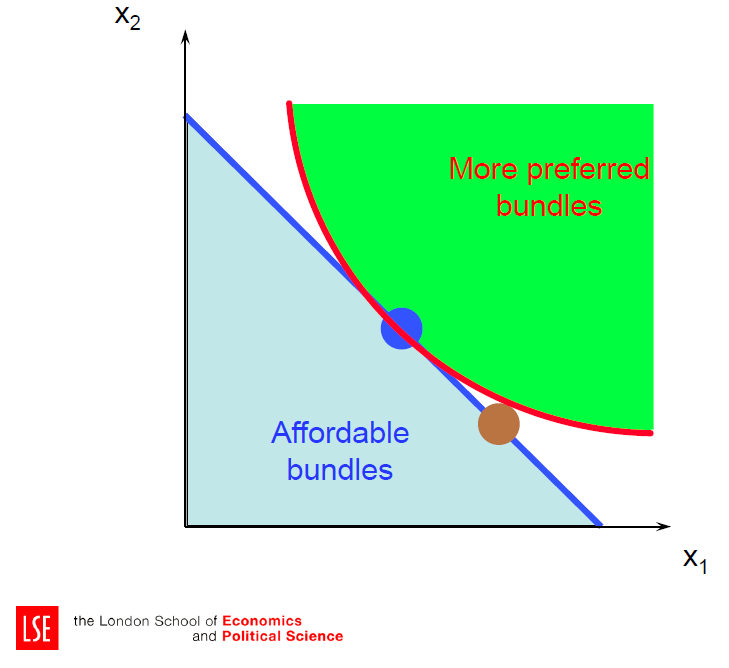# Managerial Economics Tutor in London – MG207 LSE Managerial Econ TutorWork with our Managerial Economics tutor in London for MG207 course at LSE. We have  tutors available for Microeconomics I, Microeconomics II, Principles of Microeconomics and Micro theory.

### Consumer Preferences

A consumer’s choice of what bundle to consume determines her product-level demand at the given prices.

Choice reflects preferences under constraints. We need a way to represent preferences, and then combine this information with other information about the constraints.
Analysis of these two sides of the problem allows us to derive consumer demand.

A preference relation that is complete, reflexive, and transitive can be represented by a continuous utility function. Continuity means that small changes to a consumption bundle cause only small changes to the preference level.

An indifference curve contains equally preferred bundles. Equal preference ⇒ same utility level. Therefore, all bundles in an indifference curve have the same utility level. So the bundles (4,1) and (2,2) are in the indifference curve with utility level U ≡ 4

### Utility MaximizationThe most preferred affordable bundle is called the consumer’s ORDINARY DEMAND at the given prices and budget. Ordinary demands will be denoted by x1*(p1,p2,m) and x2*(p1,p2,m). When x1* > 0 and x2* > 0 the demanded bundle is INTERIOR. the slope of the budget constraint, -p1/p2, and the slope of the indifference curve containing (x1*,x2*) are equal at (x1*,x2*).

The study of how ordinary demands x1*(p1,p2,m) and x2*(p1,p2,m) change as prices p1, p2, and income, m, change.

The curve containing all the utility-maximizing bundles traced out as p1 changes, with p2 and m constant, is the p1- price offer curve.

The plot of the x1-coordinate of the p1- price offer curve against p1 is the ordinary demand curve for commodity 1.

Other popular courses:

Get Started

## See the #1 economics mentoring platform in action

Request a demo